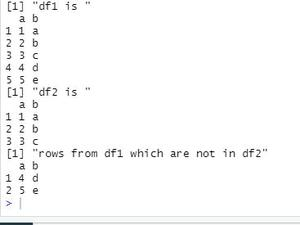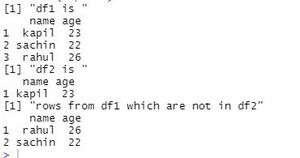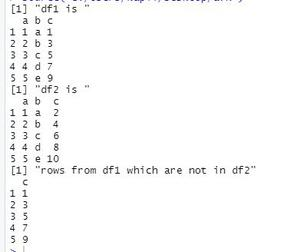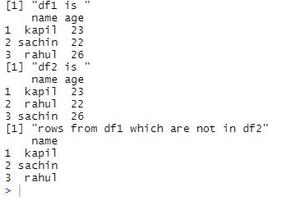GeeksforGeeks App
Open AppBrowser
Continue

# Find rows which are not in other dataframe in R

To find rows present in one dataframe that are not present in the other is known as set-difference. In this article, we will see different ways to do the same.

### Method 1: Using sqldf()

In this method simply the sql query to find set-difference is passed

Syntax:

sqldf(“sql query”)

Our query will be sqldf(‘SELECT * FROM df1 EXCEPT SELECT * FROM df2’). It will exclude all the rows from df1 that are also present in df2 and will return only rows that are only present in df1.

Example 1:

## R

 `require``(sqldf)``df1 <- ``data.frame``(a = 1:5, b=``letters``[1:5])``df2 <- ``data.frame``(a = 1:3, b=``letters``[1:3])`` ` `print``(``"df1 is "``)``print``(df1)`` ` `print``(``"df2 is "``)``print``(df2)`` ` `res <- ``sqldf``(``'SELECT * FROM df1 EXCEPT SELECT * FROM df2'``)``print``(``"rows from df1 which are not in df2"``)``print``(res)`Example 2:

## R

 `require``(sqldf)``df1 <- ``data.frame``(name = ``c``(``"kapil"``,``"sachin"``,``"rahul"``), age=``c``(23,22,26))``df2 <- ``data.frame``(name = ``c``(``"kapil"``), age = ``c``(23))`` ` `print``(``"df1 is "``)``print``(df1)`` ` `print``(``"df2 is "``)``print``(df2)`` ` `res <- ``sqldf``(``'SELECT * FROM df1 EXCEPT SELECT * FROM a2'``)``print``(``"rows from df1 which are not in df2"``)``print``(res)`### Method 2: Using setdiff()

This is an R built-in function to find the set difference of two dataframes.

Syntax:

setdiff(df1,df2)

It will return rows in df1 that are not present in df2.

Example 1:

## R

 `df1 <- ``data.frame``(a = 1:5, b=``letters``[1:5], c= ``c``(1,3,5,7,9))``df2 <- ``data.frame``(a = 1:5, b=``letters``[1:5], c = ``c``(2,4,6,8,10))`` ` `print``(``"df1 is "``)``print``(df1)`` ` `print``(``"df2 is "``)``print``(df2)`` ` `res <-``setdiff``(df1, df2)``print``(``"rows from df1 which are not in df2"``)``print``(res)`

Output:Example 2:

## R

 `df1 <- ``data.frame``(name = ``c``(``"kapil"``,``"sachin"``,``"rahul"``), age=``c``(23,22,26))``df2 <- ``data.frame``(name = ``c``(``"kapil"``,``"rahul"``, ``"sachin"``), age = ``c``(23, 22, 26))`` ` `print``(``"df1 is "``)``print``(df1)`` ` `print``(``"df2 is "``)``print``(df2)`` ` `res <- ``setdiff``(df1, df2)``print``(``"rows from df1 which are not in df2"``)``print``(res)`

Output:My Personal Notes arrow_drop_up